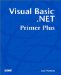# Arithmetic Operators

Simply stated, arithmetic operators are special symbols that cause Visual Basic .NET to process program expressions in a very specific manner. Table 9.1 presents the arithmetic operators that are available in Visual Basic .NET.

##### Table 9.1. Arithmetic Operators

Operator

Type

Description

*

Binary

Multiplication

/

Binary

Regular division

\

Binary

Integer division

^

Binary

Exponentiation

Mod

Binary

Modulus division

+

Binary

-

Binary

Subtraction

+

Unary

Unary plus

-

Unary

Unary minus

There may be a few surprises for you in Table 9.1. First, notice that there are two division operators. The forward-slash division operator ( / ) is the division operator that you are likely to use most often. The backward-slash division operator ( \ ) is limited to integer division and is used less often because any remainder from the division is dropped. However, integer division is very fast and may be useful to you in some limited applications.

The exponentiation operator ( ^ ) is one you've used before, at least indirectly, in the discussion of binary arithmetic in Chapter 4, "Data Types and Numeric Variables." Remember when I said 2 8 equals 256? You could produce this result in Visual Basic .NET by using the exponentiation operator with this statement:

` Result = 2 ^ 8 `

After you process this statement, Result equals 256 .

The Mod operator returns the remainder after integer division. For example, in integer division, 5 divided by 2 equals 2, with a remainder of 1. Therefore, this statement:

` Result = 5 Mod 2 `

would find Result equal to 1 , not 2 . Again, the reason is because the Mod operator returns the remainder of the division, not the quotient . A common use for the Mod operator is to determine whether a number is odd or even. In this expression:

` Result = Number Mod 2 `

Result equals 1 any time Number is an odd number, and it equals any time Number is an even number.

#### Unary Plus and Unary Minus

The unary plus ( + ) and unary minus ( - ) operators are a little strange because they are unary operators rather than binary operators. Recall from Chapter 4 that a binary operator takes two operands.

The binary arithmetic operators listed in Table 9.1 have this general form:

`  ArithmeticResult  =  Operand1 Operator Operand2  `

The unary operators, however, have this form:

`  ArithmeticResult  =  Operator Operand1  `

Notice that only a single operand is used in this expression. An example should help explain how this works. Consider the following code fragment:

` Num = 10  Result = -Num `

In this example, we are using the unary minus operator to change Num from 10 to -10 . Note that the unary minus operator works by subtracting the operand (in this case, Num ) from .

What does the following code fragment assign to Result ?

` Num = -10  Result = +Num `

If you said Result equals 10 , you are wrong! The reason is that unary plus simply returns the operand; it does not change the sign of the operand. If you need the absolute value of the number, you use the Abs() function, found in the Math library. (If you forgot about the Math library, check out how the Sqrt() function is used in Chapter 4.)Visual Basic .NET Primer Plus
ISBN: 0672324857
EAN: 2147483647
Year: 2003
Pages: 238
Authors: Jack Purdum

Similar book on Amazon